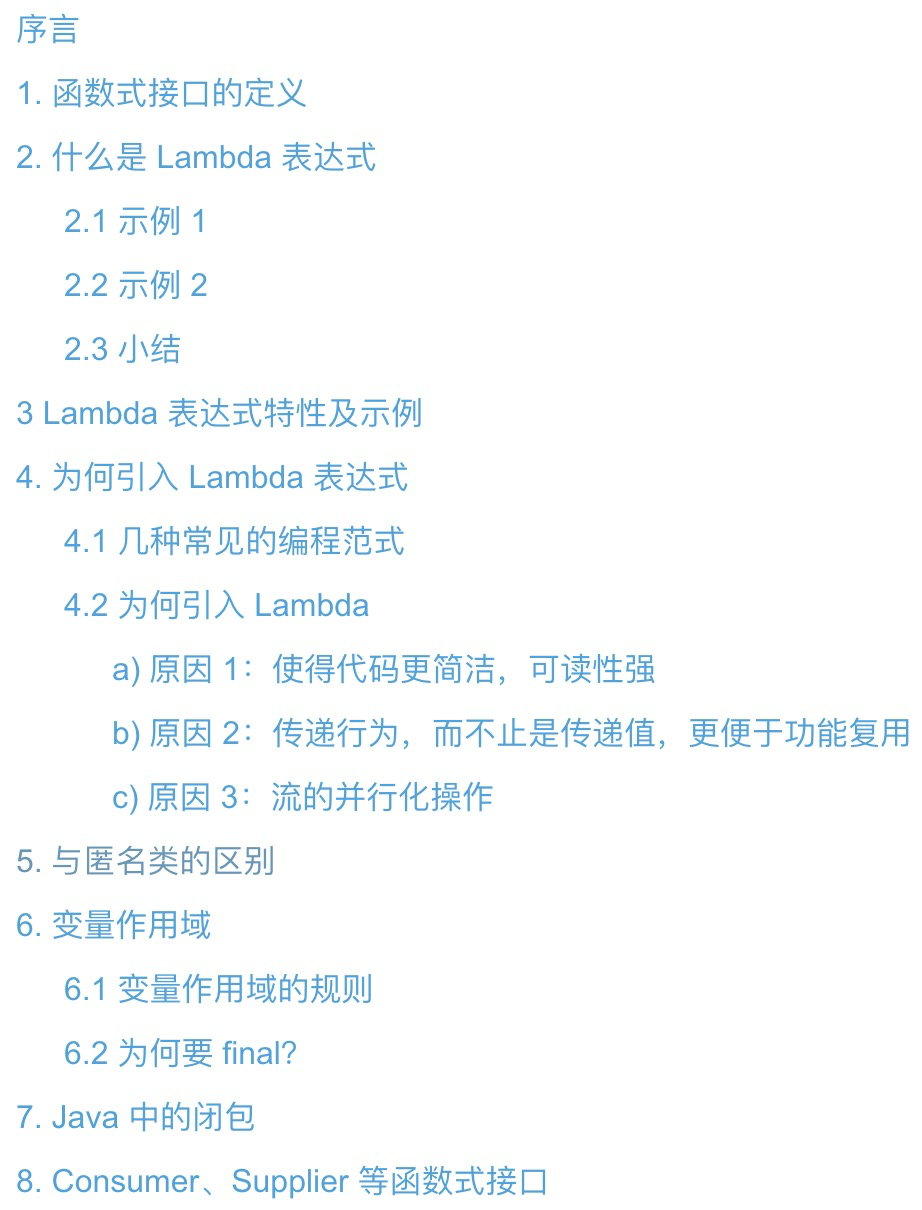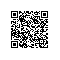# 深入探讨 Lambda 表达式（下）## 5. 与匿名类的区别

public interface DataOperate {
public boolean accept(Integer value);

public Integer convertValue(Integer value);
}

public static List<Integer> process(List<Integer> valueList, DataOperate operate) {
return valueList.stream()
.filter(value -> operate.accept(value))
.map(value -> operate.convertValue(value))
.collect(Collectors.toList());
}

public static void main(String[] args) {
List<Integer> valueList = Arrays.asList(1, 2, 3, 4, 5, 6, 7, 8, 9);

// 示例场景1: 将大于3的值翻倍，否则丢弃，得到新数组
List<Integer> newValueList1 = process(valueList, new DataOperate() {
@Override
public boolean accept(Integer value) {
return value > 3 ? true : false;
}

@Override
public Integer convertValue(Integer value) {
return value * 2;
}
});

// 示例场景2：将为偶数的值除以2，否则丢弃，得到新数组
List<Integer> newValueList2 = process(valueList, new DataOperate() {
@Override
public boolean accept(Integer value) {
return value % 2 == 0 ? true : false;
}

@Override
public Integer convertValue(Integer value) {
return value / 2;
}
});
}

## 6. 变量作用域

Variable used in lambda expression should be final or effectively final

### 6.1 变量作用域的规则

• 规则 1：局部变量不可变，域变量或静态变量是可变的

public class AClass {
private Integer num1 = 1;
private static Integer num2 = 10;

public void testA() {
int a = 1;
int b = 2;
int c = 3;
a++;
System.out.println("a=" + a); // 在 Lambda 表达式使用前有改动，编译报错
b++; // 在 Lambda 表达式中更改，报错
System.out.println("c=" + c); // 在 Lambda 表达式使用之后有改动，编译报错

System.out.println("num1=" + this.num1++); // 对象变量，或叫域变量，编译通过
AClass.num2 = AClass.num2 + 1;
System.out.println("num2=" + AClass.num2); // 静态变量，编译通过
}).start();
c++;
}
}

• 规则 2：表达式内的变量名不能与局部变量重名，域变量和静态变量不受限制

public class AClass {
private Integer num1 = 1;
private static Integer num2 = 10;

public void testA() {
int a = 1;
int a = 3; // 与外部的局部变量重名，编译报错
Integer num1 = 232; // 虽与域变量重名，允许，编译通过
Integer num2 = 11; // 虽与静态变量重名，允许，编译通过
}).start();
}
}

• 规则 3：可使用 thissuper 关键字，等同于在普通方法中使用
public class AClass extends ParentClass {
@Override
public void printHello() {
System.out.println("subClass: hello budy!");
}

@Override
public void printName(String name) {
System.out.println("subClass: name=" + name);
}

public void testA() {
this.printHello();  // 输出：subClass: hello budy!
super.printName("susu"); // 输出：ParentClass: name=susu

this.printHello();  // 输出：subClass: hello budy!
super.printName("susu"); // 输出：ParentClass: name=susu
}).start();

}
}

class ParentClass {
public void printHello() {
System.out.println("ParentClass: hello budy!");
}

public void printName(String name) {
System.out.println("ParentClass: name=" + name);
}
}

• 规则 4：不能使用接口中的默认方法（default 方法）
public class AClass implements testInterface {
public void testA() {
String name = super.getName(); // 编译报错：cannot resolve method 'getName()'
}).start();
}
}

interface testInterface {
// 默认方法
default public String getName() {
return "susu";
}
}

### 6.2 为何要 final？

• 原因 1：引入的局部变量是副本，改变不了原本的值

public static void main(String args[]) {
int a = 3;
String str = "susu";
Susu123 susu123 = (x) -> System.out.println(x * 2 + str);
susu123.print(a);
}

interface Susu123 {
void print(int x);
}

public class Java8Tester {
public Java8Tester(){
}
public static void main(java.lang.String[]){
...
}
private static void lambda$main$0(java.lang.String, int);
...
}
}

• 原因 2：局部变量存于栈中，多线程中使用有问题

public static void main(String args[]) {
int b = 1;
}

• 原因 3：线程安全问题

public void test() {
boolean flag = true;
while(flag) {
...
flag = false;
}
});
flag = false;
}

// 实例变量
private int a = 1;

public static void main(String args[]) {
Java8Tester java8Tester = new Java8Tester();
java8Tester.test();
System.out.println(java8Tester.a);

}

public void test() {
for (int i = 0; i < 10; i++) {
}
}

## 7. Java 中的闭包

function func1() {
var s1 = 32;
incre = function() {
s1 + 1;
};
return function func2(y) {
return s1 + y;
};
}

tmp = func1();
console.log(tmp(1)); // 33

incre();
console.log(tmp(1)); // 34

• 第一点，它本身是一个函数，且是一个在其他函数内部定义的函数；
• 第二点，它还携带了它作用域外的变量 s1，即外部变量

public class OuterClass {
private String name = "susu";

private class InnerClass {
private String firstName = "Shan";

public String getFullName() {
return new StringBuilder(firstName).append(" ").append(name).toString();
}

public OuterClass getOuterObj() {
// 通过 外部类.this 得到对外部环境的引用
return OuterClass.this;
}
}

public static void main(String[] args) {
OuterClass outerClass = new OuterClass();
InnerClass innerClass = outerClass.new InnerClass();
System.out.println(innerClass.getFullName());

outerClass.name = "susu1";
System.out.println(innerClass.getFullName());

System.out.println(Objects.equals(outerClass, innerClass.getOuterObj()));
}
}

#### 输出 ####
Shan susu
Shan susu1
true

public String getFullName() {
return new StringBuilder(firstName).append(" ").append(OutClass.this.name).toString();
}

OutClass.this 就是内部类持有的外部引用。

## 8. Consumer、Supplier 等函数式接口

@FunctionalInterface
public interface Consumer<T> {
/**
* Performs this operation on the given argument.
*
* @param t the input argument
*/
void accept(T t);

default Consumer<T> andThen(Consumer<? super T> after) {
Objects.requireNonNull(after);
return (T t) -> { accept(t); after.accept(t); };
}
}

• Consumer 接口：消费型函数式接口

default void forEach(Consumer<? super T> action) {
Objects.requireNonNull(action);
for (T t : this) {
action.accept(t);
}
}

public class Connection {

public Connection() {
}

public void operate() {
System.out.println("do something.");
}

public void close() {

}

public void executeTask() {
Connection conn = new Connection();
try {
conn.operate();
} catch (Exception e) {
e.printStackTrace();
} finally {
conn.close();
}
}

public class Connection {

private Connection() {

}

public void operate() {
System.out.println("do something.");
}

public void close() {

}

public static void useConnection(Consumer<Connection> consumer) {
Connection conn = new Connection();
try {
consumer.accept(conn);
} catch (Exception e) {
} finally {
conn.close();
}
}
}

Connection.useConnection(conn -> conn.operate());
• Supplier 接口：供给型函数式接口

public interface Supplier<T> {
/**
* Gets a result.
*
* @return a result
*/
T get();
}

// 示例 1
Supplier<Integer> supplier1 = () -> Integer.valueOf(32);
System.out.println(supplier1.get());  // 32

// 示例 2
Supplier<Runnable> supplier2 = () -> () -> System.out.println("abc");
supplier2.get().run(); // abc

Supplier<Runnable> supplier2 = () -> {
Runnable runnable = () -> System.out.println("abc");
return runnable;
};
supplier2.get().run();

## 小结